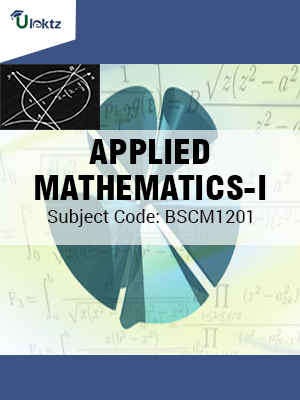•My WalletMy Order
•My Profile
•My Connections
•My Books
•My Videos
•My Tests
•My Calender
•My Messages
•My Shopping Cart
•My Orders
•Account Settings
•Help

# Book Details# APPLIED MATHEMATICS - I

 Course Code : BSCM1201 Author : uLektz University : Biju Patnaik University of Technology (BPUT) Regulation : 2010 Categories : Engineering Mathematics Format :ePUB3 (DRM Protected) Type : eBook

FREE

Description :APPLIED MATHEMATICS - I of BSCM1201 covers the latest syllabus prescribed by Biju Patnaik University of Technology (BPUT) for regulation 2010. Author: uLektz, Published by uLektz Learning Solutions Private Limited.

Note : No printed book. Only ebook. Access eBook using uLektz apps for Android, iOS and Windows Desktop PC.

##### Topics
###### UNIT-I CALCULUS

1.1 Asymptote

1.2 Curvature

1.3 Partial differentiation

1.4 Taylor’s theorem for function of two variable, Maxima and Minima for function of two variables

###### UNIT II DIFFERENTIAL EQUATION

2.1 Differential Equation: First order differential equations, Separable Equation

2.2 Exact differential equation, Linear differential equation, Bernoulli's equation and application to Electrical circuits

2.3 Linear differential equation of second and higher order, Homogeneous equation with constant co-efficient

2.4 Euler-Cauchy equations

2.5 Solution by undetermined co-efficient, Solutions by variation of parameters

2.6 Modeling of electric circuits

###### UNIT-III SERIES SOLUTION OF DIFFERENTIAL EQUATIONS

3.1 Power series method

3.2 Legendre equation and Legendre polynomials

3.3 Linear algebra, Matrices, Vectors, Determinants

3.4 System of linear equations

###### UNIT-IV EIGEN VALUES AND EIGEN VECTORS

4.1 Eigen values and eigen vectors

4.2 Symmetric and skew-symmetric matrices, Orthogonal matrices

4.3 Complex matrices, Hermitian and skew-Hermitian matrices, Unitary matrices and similarity of matrices

### Related Books